##### Actions

A finite-difference method for solving Cauchy's problem for systems of first-order differential equations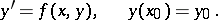In integration over a grid with a constant step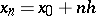the computational formulas may be based a) on extrapolation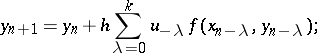or b) on interpolation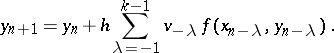For a given, the latter formula gives more accurate results but a non-linear system of equations must be solved in order to find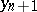.

In practical work, an approximation by the former method is found and one or two corrections are performed according to the formula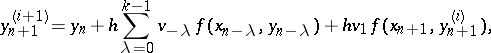which converge pointwise if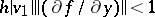. The initial conditions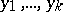for Adams' method, which are needed to begin the calculations by formula a), must be found by some special method. The error in the solution can be written as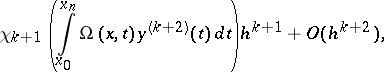where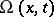is the solution of the system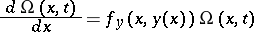when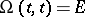.

The structure of the term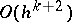is such that, for small values of, it is usually uniformly small as compared to the main term on large intervals of integration. This means that Adams' method can be applied over a large integration interval in the case of an absolutely-stable solution of the differential problem. In particular, as distinct from the Milne method, it may be used for finding stable periodic solutions of differential equations. The standard Adams procedure for integration with automatic step selection is much more involved than the standard Runge–Kutta method, since the step-changing algorithm is much more involved and the selection of the initial values of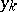is not standardized.

In the case of the equations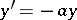,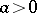, the extrapolation formula a) has the formParticular solutions of this equation are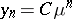, whereis a root of the equationIf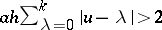, then one root of this equation is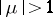, and the rounding-off errors rapidly increase. When integrating with automatic step selection, this results in an unjustified diminution of the step size. However, Adams' method proves to be more economical than the Runge–Kutta method in most cases; it was first introduced by J.C. Adams in 1855.